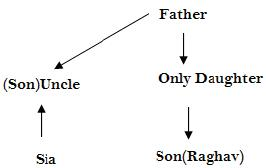Courses

# Olympiad Test: Blood Relation Test -2

## 10 Questions MCQ Test Science Olympiad Class 7 | Olympiad Test: Blood Relation Test -2

Description
This mock test of Olympiad Test: Blood Relation Test -2 for Class 7 helps you for every Class 7 entrance exam. This contains 10 Multiple Choice Questions for Class 7 Olympiad Test: Blood Relation Test -2 (mcq) to study with solutions a complete question bank. The solved questions answers in this Olympiad Test: Blood Relation Test -2 quiz give you a good mix of easy questions and tough questions. Class 7 students definitely take this Olympiad Test: Blood Relation Test -2 exercise for a better result in the exam. You can find other Olympiad Test: Blood Relation Test -2 extra questions, long questions & short questions for Class 7 on EduRev as well by searching above.
QUESTION: 1

### Sia introduced Raghav as the son of the only daughter of the father of her uncle. How is Raghav related to Sia?

Solution:

Only daughter of uncle’s father - uncle’s sister. But it is not clear that uncle’s sister is Sia’s mother.Hence, the answer is (d) cannot be determined.

QUESTION: 2

### Anirban said, “The girl is the wife of the grandson of my mother” .Who is Anirban to the girl?

Solution:

The girl is the wife of grandson of Amit’s mother i.e., the girl is the wife of son of Amit. Hence, Amit is the father-in-law of the girl.

QUESTION: 3

### Introducing a woman, Nisha said, ‘She is the daughter-in-law of the grandmother of my father’s only son.” How is the woman related to Nisha?

Solution:

My father’s only son – My brother; grandmother of my brother – My grandmother; Daughter – in – law of my grandmother – My mother.
So the lady is girl’s mother.

QUESTION: 4

If A is the mother of D, B is not the son of C, C is the father of D, D is the sister of B, then how is A related to B ?

Solution:

B is not the son of C, which means B is the daughter of C. Hence A is the mother of B since D and B are sisters only.

QUESTION: 5

Showing the lady in the park, Adarsh said, “She is the daughter of my grand-father’s only son.” How is Adarsh related to that lady?

Solution:

QUESTION: 6

Pointing to a girl Sandeep said, “She is the daughter of the only sister of my father.” How is Sandeep related to the girl?

Solution:

The girl is the daughter of the sister of Sandeep’s father. Hence, the girl is the cousin; or Sandeep is the cousin of the girl.

QUESTION: 7

Pointing to a boy in the photograph Reena said, “He is the only son of the only child of my grandfather.” How Reena is related to that boy?

Solution:

The boy in the photograph is the son of the only child of Reena’s grandfather i.e. the boy is the son of Reena’s father. Hence Reena is the sister of that boy.

QUESTION: 8

A is the son of C; C and Q are sisters; Z is the mother of Q and P is the son of Z. Which of the following statements is true?

Solution:

C and Q are sisters and A is the son of C. Hence, C is the mother of A; or Z is the mother Q.
Hence, Z is the maternal grandmother of A. P is the son of Z. Hence, P is the maternal uncle of A.

QUESTION: 9

If P + Q means P is the brother of Q; P x Q means P is the wife of Q, and P % Q means P is the daughter of Q, then which of the following means D is the uncle of A?

Solution:

None of these can be the correct option.

QUESTION: 10

P is the mother of K; K is the sister of D; D is the father of J. How is P related to J?

Solution:

P is the mother of K
K is the sister of D
D is the father of J.
Therefore, J is the nephew or niece of K and P is the grandmother of J.